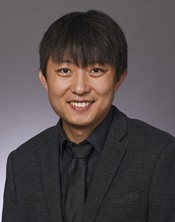×

# A New Model of Sparse Dictionary Based Material Elemental Decomposition in Multi-Energy CT for Proton Stopping Power Ratio Estimation

## C Shen , N Qin*, H Jung , M Yang , X Jia , The University of Texas Southwestern Medical Ctr, Dallas, TX

•N Qin

# Presentations

(Sunday, 7/29/2018) 1:00 PM - 1:55 PM

Room: Room 202

Purpose: Accurate calculation of stopping power ratio (SPR) is a crucial task in proton therapy treatment planning. Multi-energy CT (MECT) has shown its potential to accurately derive SPR. We previously developed a sparse dictionary-based method to derive electron density relative to water (rED) and elemental composition (EC) from MECT for SPR calculation. The optimization model employed a nonlinear forward CT model, impeding solution accuracy and computational accuracy. In this project, we propose a transformation that converts the CT model to an equivalent but linear form. It reduces complexity of the problem, improving result accuracy and efficiency.

Methods: Decomposition of rED and EC is formulated as an optimization problem that minimizes difference between measured and calculated CT numbers. We enforce the solution EC to be a sparse combination of ECs of materials in a dictionary consisting of typical tissues in human. Noticing that summation of EC for any material equals to unity, we define a new variable as a function of rED and EC to convert the forward CT model into a linear form. After solving the optimization problem with respect to the new variable, rED and EC are computed accordingly and SPR is calculated using Bethe-Bloch equation. We tested our method in both simulation and experimental studies.

Results: In simulation case, root-mean-square (RMS) SRP errors were 0.074% and 0.071% for MECT with three and four energy channels, respectively. In experiments with a Gammax phantom, RMS errors of 0.30% and 0.26% were achieved for MECT with three and four channels. These errors were substantially lower than those using the previous nonlinear model. Using the linear model also reduced computation time from 10sec to 0.075sec.

Conclusion: Introducing the variable transform effectively reduces optimization problem complexity, improving accuracy and efficiency. The proposed method is able to accurately calculate SPR using MECT.

Keywords

Not Applicable / None Entered.

Taxonomy

IM- CT: Quantitative imaging/analysis

Contact Email